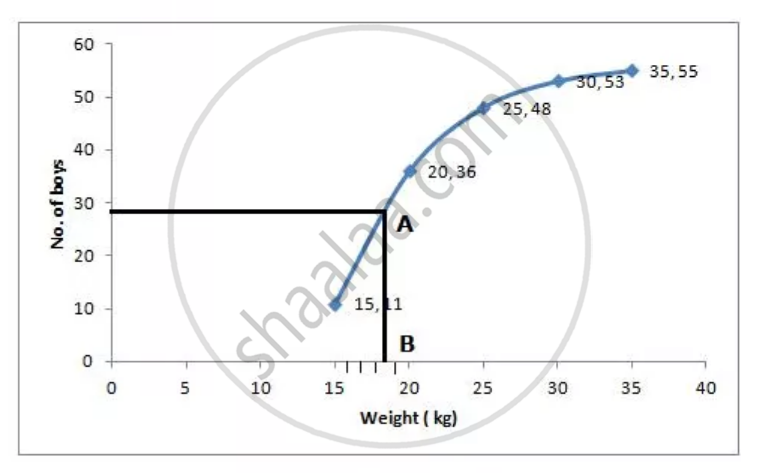Share

# By Drawing an Ogive, Estimate the Following Frequency Distribution: - ICSE Class 10 - Mathematics

ConceptMeasures of Central Tendency - Mean, Median, Mode for Raw and Arrayed Data

#### Question

By drawing an ogive, estimate the following frequency distribution:

 Weight (kg) 10-15 15-20 20-25 25-30 30-35 No.of boys 11 25 12 5 2

#### Solution

 Weight(kg) No.of boys Cumlative Frequency 10-15 11 11 15-20 25 36 20-25 12 48 25-30 5 53 30-35 2 55

Number of terms=55

∴ Median = (55+1^("th"))/2 term = 28^("th") termThrogh mark of 28 on the y-axis, draw a line parallel to x-axis which meets the curve at A. from A, draw a perpendiculer to x-axis , which meets x- axis at B

The value of B is the median which is 18.4 kg .

Is there an error in this question or solution?

#### APPEARS IN

Selina Solution for Selina ICSE Concise Mathematics for Class 10 (2019-20) (2020 to Current)
Chapter 24: Measure of Central Tendency(Mean, Median, Quartiles and Mode)
24C | Q: 8
Solution By Drawing an Ogive, Estimate the Following Frequency Distribution: Concept: Measures of Central Tendency - Mean, Median, Mode for Raw and Arrayed Data.
S